U.S. Department of Transportation
1200 New Jersey Avenue, SE
Washington, DC 20590
202-366-4000

Federal Highway Administration Research and Technology
Coordinating, Developing, and Delivering Highway Transportation Innovations

 REPORT This report is an archived publication and may contain dated technical, contact, and link information
 Publication Number:  FHWA-HRT-12-031    Date:  August 2012
 Publication Number: FHWA-HRT-12-031 Date: August 2012

# User’s Guide: Estimation of Key PCC, Base, Subbase, and Pavement Engineering Properties From Routine Tests and Physical Characteristics

PDF files can be viewed with the Acrobat® Reader®

## CHAPTER 4. RIGID PAVEMENT DESIGN FEATURES MODELS

The models developed for the prediction of MEPDG-specific inputs fall under the design features category. In developing these models, the dependent variable (e.g., deltaT for JPCP design) was determined through performing several trial and error runs of the MEPDG and establishing the optimum value that minimizes the error prediction. The independent variables were obtained from the LTPP database or MEPDG calibration files.

The MEPDG design files used to generate the dependent variable data were obtained from the model calibration performed under NCHRP 1-40D, which produced the MEPDG software program version 1.0 in 2007.(4) However, minor changes and software bug fixes have been performed since then, and the official version available at the time of this study was the MEPDG software version 1.1. Therefore, these models presented under this section are valid only for use with the distress calibration model of version 1.1 of the MEPDG software. The prediction models presented here for the estimation of design feature inputs therefore may not be valid once the products of future MEPDG updates and revisions are released.

## deltaT—JPCP Design

The equation developed to estimate the deltaT gradient variable can be expressed as follows: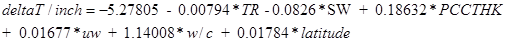Figure 79. Equation. Prediction model 15 for deltaT/inch.

Where:

deltaT/inch = Predicted average gradient through JPCP slab, °F/inch.
TR = Difference between maximum and minimum temperature for the month of construction, °F.
SW = Slab width, ft.
PCCTHK = JPCP slab thickness, inch.
uw = Unit weight of PCC used in JPCP slab, lb/ft3.
w/c = Water to cement ratio.
latitude = Latitude of the project location, degrees.

The model considers climate (TR, latitude), design (SW, PCCTHK), and material (uw, w/c) parameters. The model statistics are presented in table 28. The model was developed with 147 data points, has an R2 value of 49.67 percent, and an RMSE of 0.3199 psi. Table 29 provides details of the range of data used to develop the model.

Table 28. Regression statistics for JPCP deltaT model.

 Variable DF Estimate Standard Error t-value Pr > |t| VIF Intercept 1 -5.27805 1.06943 -4.94 < 0.0001 0 TR 1 -0.00794 0.00396 -2 0.047 1.86047 SW 1 -0.0826 0.03432 -2.41 0.0174 1.07141 PCCTHK 1 0.18632 0.0195 9.55 < 0.0001 1.0642 uw 1 0.01677 0.00669 2.51 0.0133 1.22792 w/c 1 1.14008 0.2914 3.91 0.0001 1.14857 latitude 1 0.01784 0.0072 2.48 0.0144 1.85265

Table 29. Range of data used for JPCP deltaT model.

 Parameter Minimum Maximum Average Temperature range 21.2 64.5 47.4 Slab width 12.0 14.0 12.5 PCC thickness 6.4 14.3 9.6 Unit weight 134 156 147 w/c ratio 0.27 0.72 0.46 Latitude 27.93 49.60 39.58

Figure 80 shows the predicted versus measured for the proposed JPCP deltaT gradient model, while figure 81 shows the residual errors. Note that the measured data here refers to the deltaT gradient determined by matching MEPDG prediction to field performance. Figure 82 shows the predicted versus measured deltaT for the model.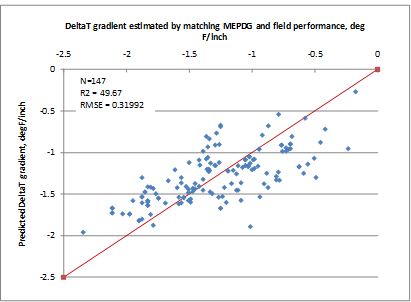Figure 80. Graph. Predicted versus measured for JPCP deltaT gradient model.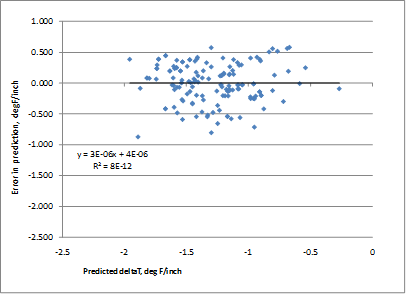Figure 81. Graph. Residual errors for JPCP deltaT gradient model.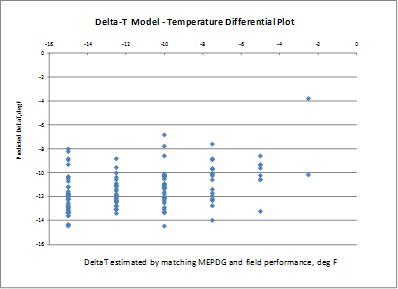Figure 82. Graph. Predicted versus measured deltaT based on the JPCP deltaT gradient model.

Figure 83 through figure 89 present the sensitivity analysis performed to examine the impact of varying the model parameters on its prediction. The parameters included are temperature range, slab width, slab thickness, unit weight, w/c ratio, and latitude. For each sensitivity analysis, the variable of interest was varied while holding all other variables constant at their typical values. Typical values used in this analysis were 24 °F temperature range, 12-ft slab width, 10-inch slab thickness, 145 lb/ft3 unit weight, 0.40 w/c ratio, and 40 degrees latitude.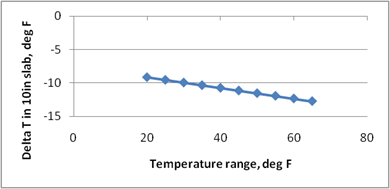Figure 83. Graph. Sensitivity of predicted deltaT to temperature range during month of construction.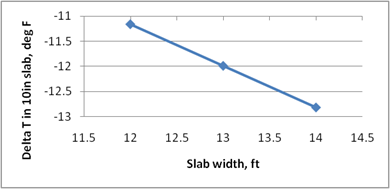Figure 84. Graph. Sensitivity of predicted deltaT to slab width.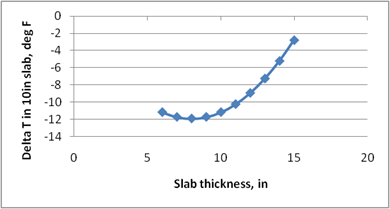Figure 85. Graph. Sensitivity of predicted deltaT to slab thickness.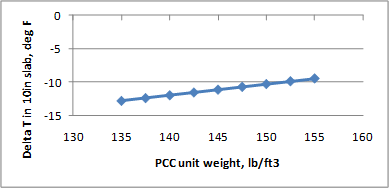Figure 86. Graph. Sensitivity of predicted deltaT to PCC slab unit weight.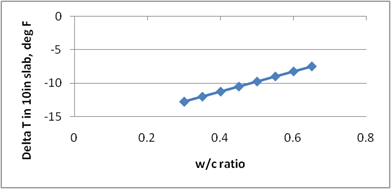Figure 87. Graph. Sensitivity of predicted deltaT to PCC w/c ratio.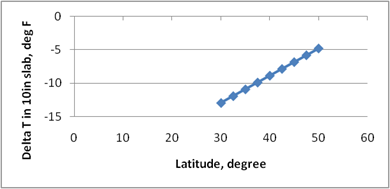Figure 88. Graph. Sensitivity of predicted deltaT to latitude of the project location.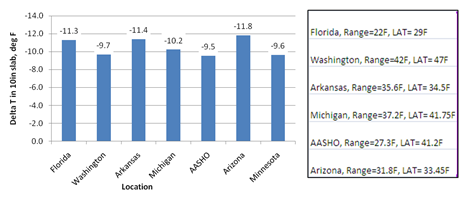Figure 89. Graph. Predicted deltaT for different locations in the United States.

The following are brief observations from these sensitivity analyses:

• For the typical values used for each of these variables, the deltaT gradients estimated are in a reasonable range.
• An increase in local climate temperature range increases the temperature gradient (see figure 83). The local climate temperature range is indicative of the level of temperature drop the project location can experience. The larger the difference in the temperature between day and night (assuming paving is performed in the daytime), the larger the negative temperature gradient locked into the slab as the slab hardens within a 24-h period.
• Wider slabs produce a larger built-in gradient (see figure 84), as has been validated in several field studies. The total thermal expansion is larger for a longer/wider slab; therefore, the resulting curvature of the slab induces a greater lift-up at the slab corners. The data did not show a significant effect of the slab length or joint spacing parameter.
• Thicker slabs reduce the deltaT gradient, as shown in figure 85. This is the expected trend, as thicker slabs (due to a greater weight) tend to restrain the corners from curling up as the concrete hardens. This figure also shows that for very thin slabs (< 8 inches), the effect is reversed. The physical significance of this cannot be fully explained or supported with data. Therefore, it is necessary to evaluate the sensitivity to each parameter while selecting deltaT for each project.
• The larger unit weight of the PCC material used in the JPCP slab also reduces the magnitude of built-in gradient (see figure 86) primarily because of the restraint provided by the heavier slab during hardening.
• Lower w/c ratios have a higher rate of hydration; therefore, the PCC slab remains plastic for a shorter duration of time. Strength gain offers the slab the rigidity necessary to bear against the base and does not allow the slab corners to curl up. Therefore, lower w/c ratios tend to have higher built-in gradients, as seen in figure 87. Furthermore, at very low w/c ratios, the PCC mix undergoes autogeneous shrinkage, which increases the potential for higher gradients in the slab.
• Figure 88 and figure 89 show the effect of latitude on predicted deltaT gradients. The United States lies between 30 and 50 degrees latitude in the Northern Hemisphere. The full range of latitudes is covered in figure 88. While this plot might appear to show deltaT’s high degree of sensitivity to the latitude parameter, for routine predictions using this model, the temperature range is a critical input. In other words, a given maximum temperature in the southern United States could have a much different temperature range relative to a location in the northern United States with the same maximum temperature. Therefore, the latitude parameter has to be evaluated combined with the temperature range parameter as shown in figure 89. The predicted deltaT for several locations in the United States are presented.

### Using the JPCP deltaT Model

This section provides an example for the use of the JPCP deltaT model developed under this study. The section used to describe the process is the LTPP Specific Pavement Studies 2 section 04_0213 located in Maricopa County, AZ, and constructed in July 1993. The following latitude, design, and material inputs required for the deltaT prediction model can be obtained from the MEPDG inputs:

• Latitude: 33.45 degrees north.
• PCC thickness: 8.3 inches.
• Slab width: 14 ft.
• PCC unit weight: 145.3 lb/ft3.
• PCC w/c ratio: 0.365.

The temperature range input to this model is the difference between the mean monthly maximum and minimum temperatures for the month of July from historical climate data records (as climate data included in the MEPDG). If the user does not have this information readily available, the data to compute the temperature range can be determined from the output file of the MEPDG analysis of this section. The output file (i.e., titled “04_0213.xls”) contains a worksheet titled “Climate” with key climate data for the specific location (or the virtual climate station created). This worksheet includes the monthly climate summary with minimum and maximum temperature by month for all years of data used under the headings “Min. Temp. (°F)” and “Max. Temp. (°F),” respectively. (Note that this summary also includes “Average Temp. (°F),” “Max. Range (°F),” “Precip. (in.),” “Average Wind (mph),” “Average Sun (%),” “Number Wet Days,” and “Max. Frost (in.).” However, these data are not of relevance to the deltaT model.

For the month of July, the average minimum and maximum temperatures are 73 and 111.7 °F, respectively. The difference between these temperatures is 38.7 °F.

Using these inputs, the deltaT gradient can be calculated as -1.7457138 °F/inch. For the slab thickness of 8.3 inches, this is equivalent to a deltaT of -14.5 °F. This value is significantly higher than the default -10 °F/inch. This input can be revised in an MEPDG file and reanalyzed to evaluate the predicted transverse cracking performance.

## deltaT—CRCP Design

The equation developed to estimate the CRCP deltaT gradient variable is as follows:Figure 90. Equation. Prediction model 16 for deltaT/inch.

Where:

deltaT/inch = Predicted gradient in CRCP slab, °F/inch.
MaxTemp = Maximum temperature for the month of construction, °F.
MaxTempRange = Maximum temperature range for the month of construction, °F.
PCCTHK = JPCP slab thickness, inch.
Chert = 1 if PCC mix coarse aggregate is chert, or 0 if otherwise.
Granite = 1 if PCC mix coarse aggregate is granite, or 0 if otherwise.
Limestone = 1 if PCC mix coarse aggregate is limestone, or 0 if otherwise.
Quartzite = 1 if PCC mix coarse aggregate is quartzite, or 0 if otherwise.

The model considers climate (MaxTemp and MaxTempRange), design parameters (PCCTHK), and material (Aggregate type) parameters. The model statistics are presented in table 30. The model was developed with 35 data points, has an R2 value of 82.5 percent, and an RMSE of 0.27932 psi. Table 31 provides details of the range of data used to develop the model.

Table 30. Regression statistics for CRCP deltaT model.

 Variable DF Estimate Standard Error t-Value Pr > |t| VIF Intercept 1 12.93007 1.98459 6.52 < 0.0001 0 MaxTemp 1 -0.15101 0.01793 -8.42 < 0.0001 3.46347 MaxTempRange 1 -0.10241 0.01869 -5.48 < 0.0001 2.00933 Chert 1 3.279 0.30508 10.75 < 0.0001 2.24965 Granite 1 1.55013 0.22656 6.84 < 0.0001 4.96262 Limestone 1 1.40009 0.18956 7.39 < 0.0001 4.00053 Quartzite 1 2.01838 0.39449 5.12 < 0.0001 1.93773 PCCTHK 1 0.11299 0.0705 1.6 0.1207 1.68624

Table 31. Range of data used for CRCP deltaT model.

 Parameter Minimum Maximum Average Maximum temperature 78.4 99.2 90.3 Temperature range 24.8 40.4 30.4 Chert 0 1 0.06 Granite 0 1 0.31 Limestone 0 1 0.46 Quartzite 0 1 0.03 PCC thickness 5.6 9.5 8.4

Figure 91 shows the predicted versus measured for the proposed CRCP deltaT gradient model, while figure 92 shows the residual errors. Note that the measured data here refers to the deltaT gradient determined by matching MEPDG prediction to field performance.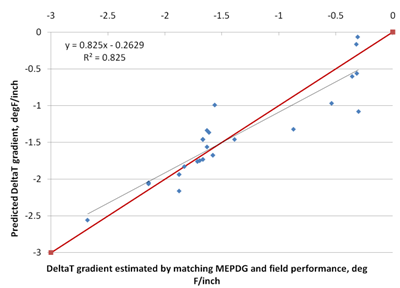Figure 91. Graph. Predicted versus measured for CRCP deltaT model.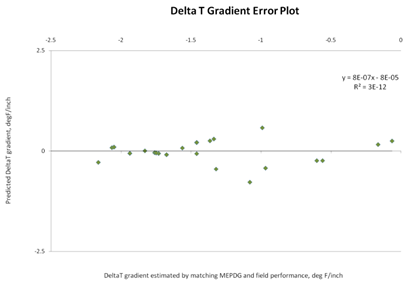Figure 92. Graph. Residual errors for CRCP deltaT model.

Figure 93 through figure 96 show the sensitivity of the deltaT differential calculation to the parameters maximum temperature of the project location, maximum temperature range, CRCP slab thickness, and geographic location, respectively. The trends observed in the model—CRCP deltaT increasing with increasing maximum temperature and increasing temperature range— are reasonable. While the effect of slab thickness shows a linear relationship with the deltaT gradient, the magnitude of the coefficient for this variable results causes the deltaT differential (CRCP deltaT gradient × thickness) to assume a nonlinear relationship with the deltaT differential, peaking at about 10 inches. Figure 96 shows the deltaT predictions for projects selected from LTPP sites in Texas, Illinois, Virginia, Mississippi, Oregon, and Georgia.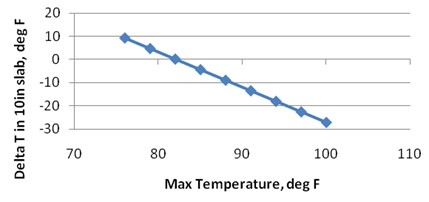Figure 93. Graph. Effect of maximum temperature on CRCP deltaT prediction model.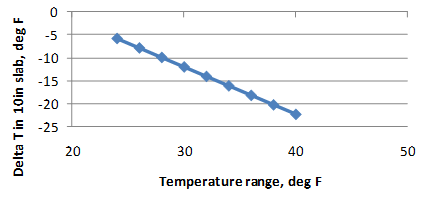Figure 94. Graph. Effect of temperature range on CRCP deltaT prediction model.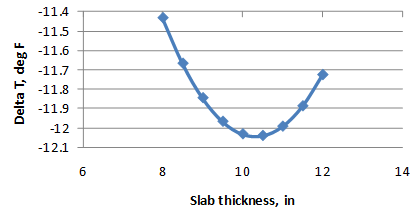Figure 95. Graph. Effect of slab thickness on CRCP deltaT prediction model.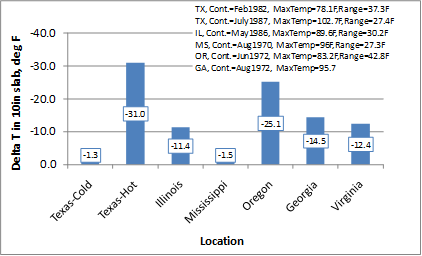Figure 96. Graph. Effect of geographic location on CRCP deltaT prediction model.

The sensitivity analyses show reasonable trends but do not demonstrate that the model is robust. It is not clear, from an engineering standpoint, if the range of predicted values and their magnitudes are practical and realistic. The wide range of deltaT has a significant effect on design thickness. The data used to develop the model show very strong correlations, and it is likely that the predictions are valid, at least within a certain range of inputs. The current analyses and the data available are not adequate to determine these ranges. It is therefore recommended that this model be used with extreme caution.

### Using the CRCP deltaT Model

The CRCP deltaT model shares similarities with the JPCP deltaT model. The section used to describe the process is the LTPP General Pavement Studies section in Illinois, 17_5020, which was constructed in May 1986. The CRCP thickness is 8.6 inches, and the PCC mix used a limestone aggregate. The following inputs can be directly obtained from the MEPDG input file:

• PCCTHK: 8.6 inches.
• Chert: 0.
• Granite: 0.
• Limestone: 1.
• Quartzite: 0.

The maximum temperature and maximum temperature range can be obtained by running the design file and deriving this input from the worksheet titled “Climate.” For the month of May, the maximum temperature and maximum temperature range for this location were 89.6 and 39.2 °F, respectively. Using these inputs, the CRCP deltaT gradient can be calculated as -1.3214 °F/inch. For the slab thickness of 8.6 inches, this is equivalent to a deltaT of -11.36 °F. This value is comparable to the -10-°F default. This input can be revised in an MEPDG file and reanalyzed to predict punchout development over time.

Federal Highway Administration | 1200 New Jersey Avenue, SE | Washington, DC 20590 | 202-366-4000
Turner-Fairbank Highway Research Center | 6300 Georgetown Pike | McLean, VA | 22101